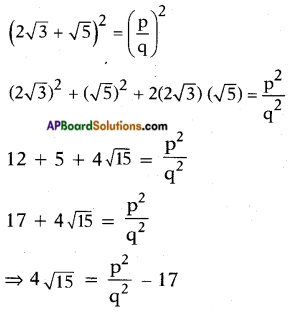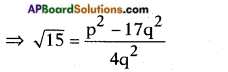AP State Board Syllabus AP SSC 10th Class Maths Textbook Solutions Chapter 1 Real Numbers Optional Exercise Textbook Questions and Answers.

## AP State Syllabus SSC 10th Class Maths Solutions 1st Lesson Real Numbers Optional Exercise

### 10th Class Maths 1st Lesson Real Numbers Optional Exercise Textbook Questions and Answers

Question 1.
Can the number 6n, n being a natural number, end with the digit 5? Give reason.
Given number = 6n ; n ∈ N
6n to be end in 5; it should be divisible by 5
6n = (2 × 3)n
The prime factors of 6n are 2 and 3.
It can’t end with the digit 5.Question 2.
Is 7 × 5 × 3 × 2 + 3 a composite number? Justify your answer.
Given:
7 × 5 × 3 × 2 + 3
= 3 (7 × 5 × 2 + 1)
= 3 × (70 + 1)
= 3 × 71
∴ The given number has two factors namely 3 and 71.
Hence it is a composite number.

Question 3.
Prove that (2√3 + √5 ) is an irrational number. Also check whether (2√3 + √5) (2√3 – √5) is rational or irrational.
To prove:
2√3 + √5 is an irrational number. On contrary, let us suppose that 2√3 + √5 be a rational number.
Then 2√3 + √5 = $$\frac{p}{q}$$
Squaring on both sides, we getL.H.S = an irrational number.
R.H.S = p, q being integers, $$\frac{p^{2}-17 q^{2}}{4 q^{2}}$$ is a rational number.
This is a contradiction to the fact that √l5 is an irrational. This is due to our assumption that 2√3 + √5 is a rational. Hence our assumption is wrong and 2√3 + √5 is an irrational number. Also,
(2√3 + √5) (2√3 – √5)
= (2√3)2 – (√5)2
[∵ (a + b) (a – b) = a2 – b2]
= 4 × 3 – 5
= 12 – 5 = 7, a rational number.Question 4.
If x2 + y2 = 6xy, prove that 2 log (x + y) = log x + log y + 3 log 2.
Given: x2 + y2 = 6xy
x2 + y2 + 2xy = 6xy + 2xy
(x + y)2 = 8xy
Taking logarithms on both sides log (x + y)2 = log8xy
⇒ 2log(x + y)= log8 + logx + logy [∵ logxm = mlogx]
[∵ logxy = logx + logy]
= log23 + logx + logy
⇒ 2log(x + y) = logx + logy + 3log2

Question 5.
Find the number of digits in 42013, if log102 = 0.3010.